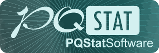#PQStat - Baza Wiedzy

### Pasek boczny

en:statpqpl:porown3grpl:nparpl:anova_chipl

### The Chi-square test for multidimensional contingency tables

Basic assumptions:

Hypotheses:where:andobserved frequencies in a contingency table and the corresponding expected frequencies.

The test statistic is defined by:This statistic asymptotically (for large expected frequencies) has the Chi-square distribution with a number of degrees of freedom calculated using the formula:- for 3-dimensional tables.

The p-value, designated on the basis of the test statistic, is compared with the significance level:The settings window with the Chi-square (multidimensional) test can be opened in Statistics menu → NonParametric tests (unordered categories)Chi-square (multidimensional) or in ''Wizard''.Note

This test can be calculated only on the basis of raw data.

1)
Cochran W.G. (1952), The chi-square goodness-of-fit test. Annals of Mathematical Statistics, 23, 315-345
en/statpqpl/porown3grpl/nparpl/anova_chipl.txt · ostatnio zmienione: 2022/02/13 17:25 przez admin

### Narzędzia strony